# Tiles

It is given an area of 5m x 4m
One tile is 40 x 40 cm
How many tiles are needed in an area of 5 m x 4 m?
And how many tiles need to be cut (if it not possible for the tiles to fall exactly)?

Result

n =  125
x =  5

#### Solution:Leave us a comment of example and its solution (i.e. if it is still somewhat unclear...):Be the first to comment!## Next similar examples:

1. On the farm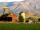The farm took 221 tons of potatoes from 17 hectares. How many tons of potatoes taken from one hectare?
2. AreaCalculate: ?
3. Rectangle A2dim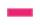Calculate the side of the rectangle, if you know that its area is of 2590 m2 and one side is 74 m.
4. Crates 2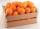One crate will hold 50 oranges. If Bob needs to ship 932 oranges, how many crates will he need?
5. Apples 2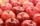How many minimum apples are in the cart, if possible is completely divided into packages of 6, 14 and 21 apples?
6. Troops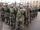If the general sorts troops into the crowd by nine left 6. How many soldiers has regiment if we know that they are less than 300?
7. BiketrialKamil was biketrial. Before hill he set the forward gear with 42 teeth and the back with 35 teeth. After how many exercises (rotation) of the front wheel both wheels reach the same position?
8. The resultHow many times I decrease the number 1632 to get the result 24?
9. Math classificationIn 3A class are 27 students. One-third got a B in math and the rest got A. How many students received a B in math?
10. Divisibility 2How many divisors has integer number 13?
11. Three Titanics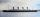Three steamers sailed from the same port on the same day. The first came back on the third day, fourth 4th day and the third returned sixth day. How many days after leaving the steamers met again in the harbor?
12. Sweets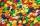Mom bought box of sweets for their children.Whole package of 100 sweets divided among 4 their children so that each child receives the most and for she remains the least sweets. How many sweets left for mom.
13. Fractions 4How many 2/3s are in 6?
14. Date game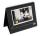Let now is Wednesday. What day is after 248 days? Write the result as a number: 1=Monday, 2=Tuesday, 3=Wednesday, 4=Thursday, 5=Friday, 6=Saturday, 7=Sunday?
15. Pizza 5You have 2/4 of a pizza and you want to share it equally between 2 people how much pizza does each person get?
16. Street numbersLada came to aunt. On the way he noticed that the houses on the left side of the street have odd numbers on the right side and even numbers. The street where he lives aunt, there are 5 houses with an even number, which contains at least one digit number 6.
17. Decomposition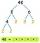Make decomposition using prime numbers of number 155. Result write as prime factors (all, even multiple)